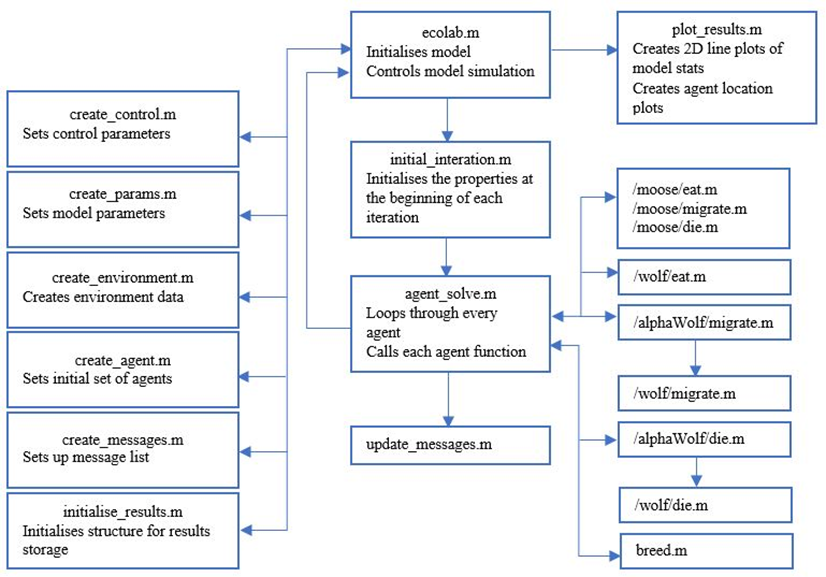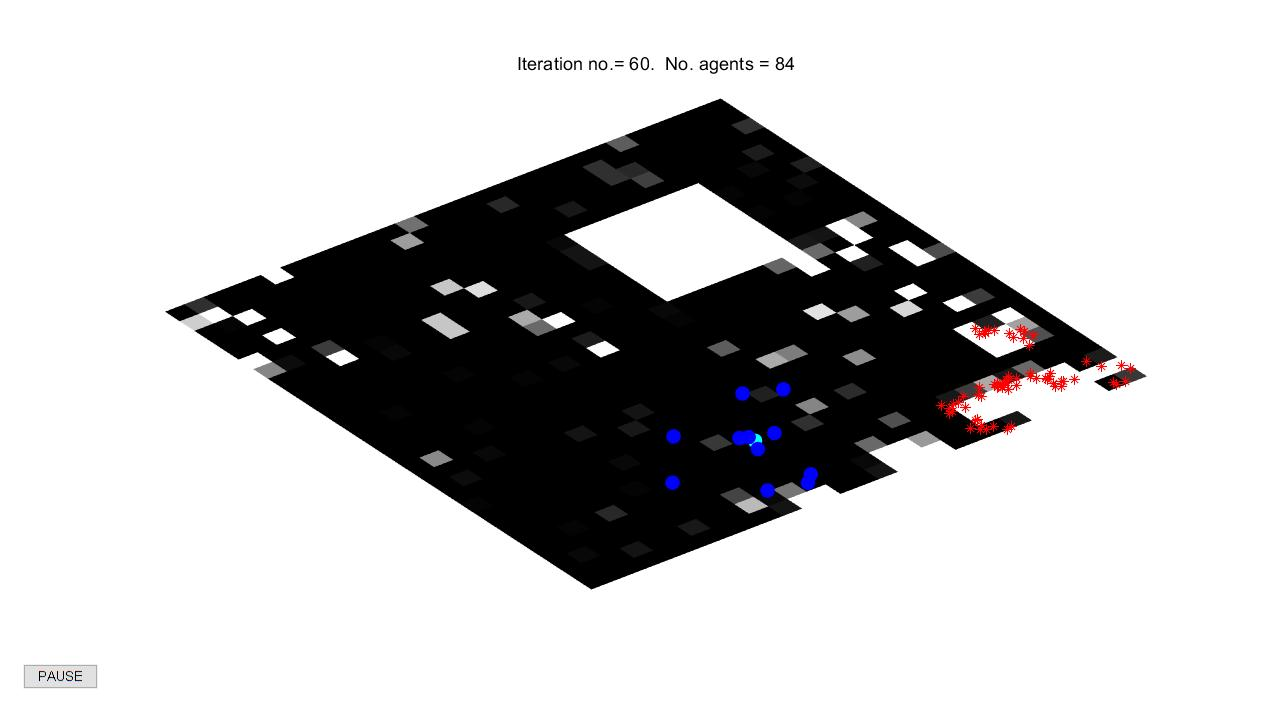生态系统建模

GitHub项目地址：nature-system

Agent指个体，Agent-base model即基于个体的模型。在Agent-based model中，虚拟实体(virtual entity)和现实个体一一对应。一般来说，虚拟实体的属性是对现实个体的抽象，虚拟实体的行为则是依照已经写好的规则迭代。这种建模方式往往涉及个体详细的行为模式，因此适合以研究个体行为规则为目的的建模。

Note: 除了Agent-base model之外，Equation-based model也是一种比较常见的建模方式。Agent-based model适合表达每个个体身上发生了什么，比如个体的速度、位置的变化等。Equation-based model则而更适合表达系统平均发生了什么，比如人口模型中人口数量变化就更适合用Equation-based model建模。

模型假设

（一）生物的属性

# age food pos speed last_breed population packNo range eaten

（二）生物的行为

1.死亡(die)

2.生育(breed)

3.迁徙(migrate)

PS: 本模型有地理范围限制，所有生物均不可以移动到地图之外的地方。因此在代码实现时，所有生物在迁徙之前，都必须验证移动位置的有效性。

4.捕猎(eat)

pk = 1 - (d/spd)
if pk > rand
{麋鹿死，狼食物加1，狼移动到麋鹿原先的位置}
end

整体架构代码实现

1.下面这段代码用于维护各生物的属性数据，每轮迭代前都要执行。

function [agent] = initial_iteration(agent,nf)
% This a new function to initial the property at the beginning of each iteration

global MESSAGES PARAM

%% initial alphaWolf.eaten at the beginning of each iteration
%eaten is the number of moose be eaten at each iteration, this
%number will be useful to decision making process of migrate.
for p = 1:length(agent)
if isa(agent{p},'alphaWolf')
agent{p}.eaten = 0;
end
end

%% initial wolf.migration at the beginning of each iteration
% wolf.migration
for q = 1:length(agent)
if isa(agent{q},'wolf')
agent{q}.migration = 0;
end
end

%% update the alphaWolf.population of wolf pack for each iteration
for m = 1:length(agent)
currAgent = agent{m};
if isa(currAgent,'alphaWolf')
count = 0;
for n = 1:length(agent)
if isa(agent{n},'wolf') & agent{n}.packNo == m & MESSAGES.atype(n) ~= 0
count = count + 1;
end
end
currAgent.population = count;
agent{m} = currAgent;
end
end

%% updatet alphaWolf.range at the beginning of each iteration
for m = 1:length(agent)
currAgent = agent{m};
if isa(currAgent,'alphaWolf')
if currAgent.population <= PARAM.F_SIZE
nrange = ceil(PARAM.F_SPD/2 * (1 + nf/5));
else
nrange = PARAM.F_SPD;
end
currAgent.range = nrange;
agent{m} = currAgent;
end
end

if isa(agent{p},'alphaWolf')

if agent{n}.packNo == m

2.下面这段代码出自从agent_solve中。

for cn=n:-1:1
curr=agent{cn};
if isa(curr,'moose')|isa(curr,'wolf')|isa(curr,'alphaWolf')
%% eat rules
% moose
if isa(curr,'moose')
[curr,moose_eaten]=eat(curr,cn);
end
% wolf
if isa(curr,'wolf')        %alphaWolf can not chase prey
[curr,agent]=eat(curr,cn,agent);  %eating rules (mooses eat food, wolves eat mooses)
end
if isa(curr,'alphaWolf')
eaten=curr.eaten;
end

%% migrate rule
% if population is large, wolf pack will be active
flag = 0;
if isa(curr,'alphaWolf') & curr.population > PARAM.F_SIZE & eaten < ceil(curr.population * 1/2)
[curr,agent_migrate]=migrate(curr,cn,agent,eaten);              % if no food was eaten, then migrate in search of some
agent = agent_migrate;                      %up date cell array with modified agent data structure
flag =1;
% if population is small, wolf pack will not move so frequently
elseif isa(curr,'alphaWolf') & curr.population <= PARAM.F_SIZE & eaten < ceil(curr.population * 1/4)
[curr,agent_migrate]=migrate(curr,cn,agent,eaten);              % if no food was eaten, then migrate in search of some
agent = agent_migrate;                      %up date cell array with modified agent data structure
flag =1;
elseif isa(curr,'moose') & moose_eaten==0
curr=migrate(curr,cn);
end
% if the alphawolf did not migrate,flag is 0
% in this case,wolf should move randomly to find the food
if isa(curr,'alphaWolf') & flag == 0
for p = 1:length(agent)
if isa(agent{p},'wolf') & agent{p}.packNo == cn & MESSAGES.atype(p) ~= 0 & agent{p}.migration == 0
[agent{p}] = migrate(agent{p},p,agent);
end
end
end

%% death rule
% wolf (from old age)
% moose (from starvation or old age)
if isa(curr,'moose')|isa(curr,'wolf')
[curr,klld]=die(curr,cn);
end

% alphaWolf (from old age) and wolf pack (from starvation)
% PS: alphaWolf represent for the whole wolf pack here
if isa(curr,'alphaWolf')
[curr,klld,agent]=die(curr,cn,agent);
end

%% breed rule
% moose
if isa(curr,'moose')&klld==0
new=[];
[curr,new]=breed(curr,cn);			%breeding rule
if ~isempty(new)					%if current agent has bred during this iteration
n_new=n_new+1;                 %increase new agent number
agent{n+n_new}=new;			%add new to end of agent list
end
end

% alphaWolf
% only alphaWolf can breed, wolf can not
if isa(curr,'alphaWolf')&klld==0
new=[];
[curr,new]=breed(curr,cn);			%breeding rule
if ~isempty(new)					%if current agent has bred during this iteration
for ite = 1:length(new)
n_new=n_new+1;                 %increase new agent number
agent{n+n_new}=new{ite};			%add new to end of agent list
end
end
end
agent{cn}=curr;                          %up date cell array with modified agent data structure
end
end

for cn=n:-1:1

运行结果